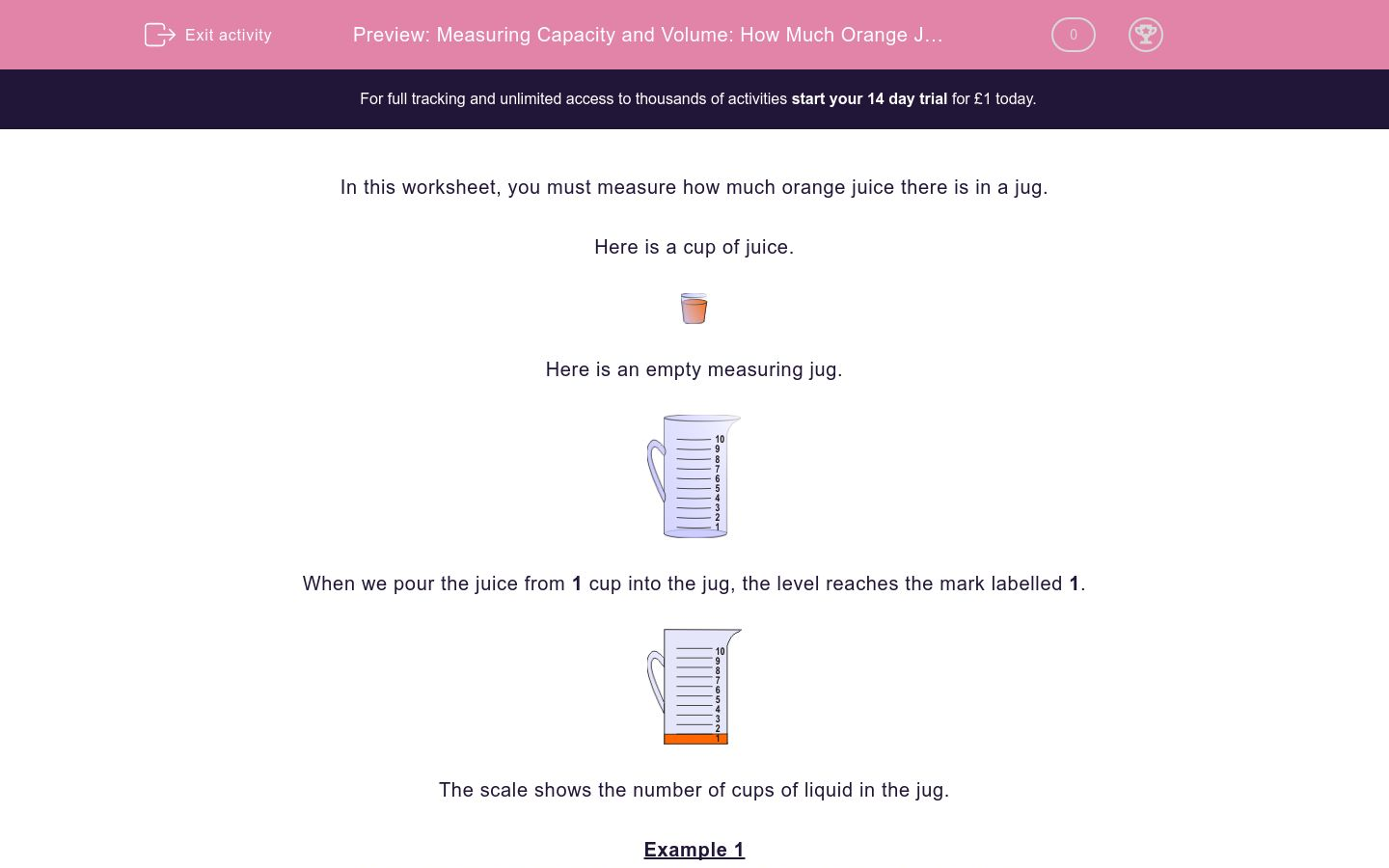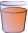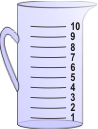# Measuring Capacity and Volume: How Much Orange Juice?

In this worksheet, students read the capacity of liquid in cups shown on the scale of a jug.Key stage:  KS 2

Curriculum topic:   Maths and Numerical Reasoning

Difficulty level:### QUESTION 1 of 10

In this worksheet, you must measure how much orange juice there is in a jug.

Here is a cup of juice.Here is an empty measuring jug.When we pour the juice from 1 cup into the jug, the level reaches the mark labelled 1.The scale shows the number of cups of liquid in the jug.

Example 1

Read the scale to find out how many cups of orange juice are in the jug.The juice reaches the 6 mark, so we will get 6 cups of orange juice.Example 2

Read the scale to find out how many more cups of orange juice are needed to reach the 10 mark.The juice reaches the 6 mark.

10 - 6 = 4.

We need 4 more cups to reach the 10 mark.Read the scale to find out how many cups of orange juice are in the jug.Read the scale to find out how many cups of orange juice are in the jug.Read the scale to find out how many more cups of orange juice are needed to reach the 10 mark.Read the scale to find out how many cups of orange juice are in the jug.Read the scale to find out how many more cups of orange juice are needed to reach the 10 mark.Read the scale to find out how many cups of orange juice are in the jug.Read the scale to find out how many cups of orange juice are in the jug.Read the scale to find out how many cups of orange juice are in the jug.Read the scale to find out how many cups of orange juice are in the jug.Read the scale to find out how many more cups of orange juice are needed to reach the 10 mark.• Question 1

Read the scale to find out how many cups of orange juice are in the jug.2
EDDIE SAYS
The juice reaches the 2 mark.
• Question 2

Read the scale to find out how many cups of orange juice are in the jug.7
EDDIE SAYS
The juice reaches the 7 mark.
• Question 3

Read the scale to find out how many more cups of orange juice are needed to reach the 10 mark.7
EDDIE SAYS
The juice reaches the 3 mark.
10 - 3 = 7.
• Question 4

Read the scale to find out how many cups of orange juice are in the jug.6
EDDIE SAYS
The juice reaches the 6 mark.
• Question 5

Read the scale to find out how many more cups of orange juice are needed to reach the 10 mark.6
EDDIE SAYS
The juice reaches the 4 mark.
10 - 4 = 6.
• Question 6

Read the scale to find out how many cups of orange juice are in the jug.1
EDDIE SAYS
The juice reaches the 1 mark.
• Question 7

Read the scale to find out how many cups of orange juice are in the jug.5
EDDIE SAYS
The juice reaches the 5 mark.
• Question 8

Read the scale to find out how many cups of orange juice are in the jug.3
EDDIE SAYS
The juice reaches the 3 mark.
• Question 9

Read the scale to find out how many cups of orange juice are in the jug.4
EDDIE SAYS
The juice reaches the 4 mark.
• Question 10

Read the scale to find out how many more cups of orange juice are needed to reach the 10 mark.5
EDDIE SAYS
The juice reaches the 5 mark.
10 - 5 = 5
---- OR ----